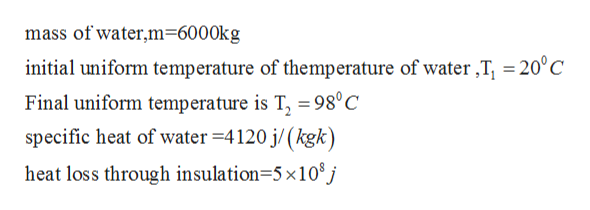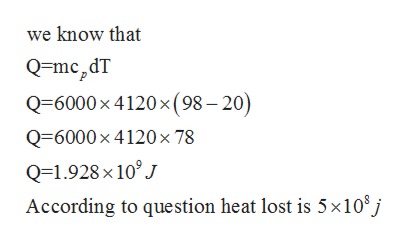# A 6000 Kg mass of water is brought to a uniform temperature of 98 ˚C in a heavily insulated tank using an electrical heater. The initial uniform temperature of the water is 20 ˚C. The heat loss through the insulation is 5(108) J as the mass of water is heated. The specific heat of water is 4,120 J/(Kg K). Calculate the electrical energy required to perform this process.

Question
199 views

A 6000 Kg mass of water is brought to a uniform temperature of 98 ˚C in a heavily insulated tank using an electrical heater. The initial uniform temperature of the water is 20 ˚C. The heat loss through the insulation is 5(108) J as the mass of water is heated. The specific heat of water is 4,120 J/(Kg K). Calculate the electrical energy required to perform this process.

check_circle

Step 1

Given data as per questionhelp_outlineImage Transcriptionclosemass of water,m=6000kg initial uniform temperature of themperature of water,T, = 20°C Final uniform temperature is T, =98°C specific heat of water 4120 j/(kgk heat loss through insulation=5x108j fullscreen
Step 2help_outlineImage Transcriptionclosewe know that Q=mc2dT Q=6000x 4120x (98-20) Q=6000 x 4120 x 78 Q=1.928 x 10°J According to question heat lost is 5x10°j fullscreen
Step 3

### Want to see the full answer?

See Solution

#### Want to see this answer and more?

Solutions are written by subject experts who are available 24/7. Questions are typically answered within 1 hour.*

See Solution
*Response times may vary by subject and question.
Tagged in

### Thermodynamics